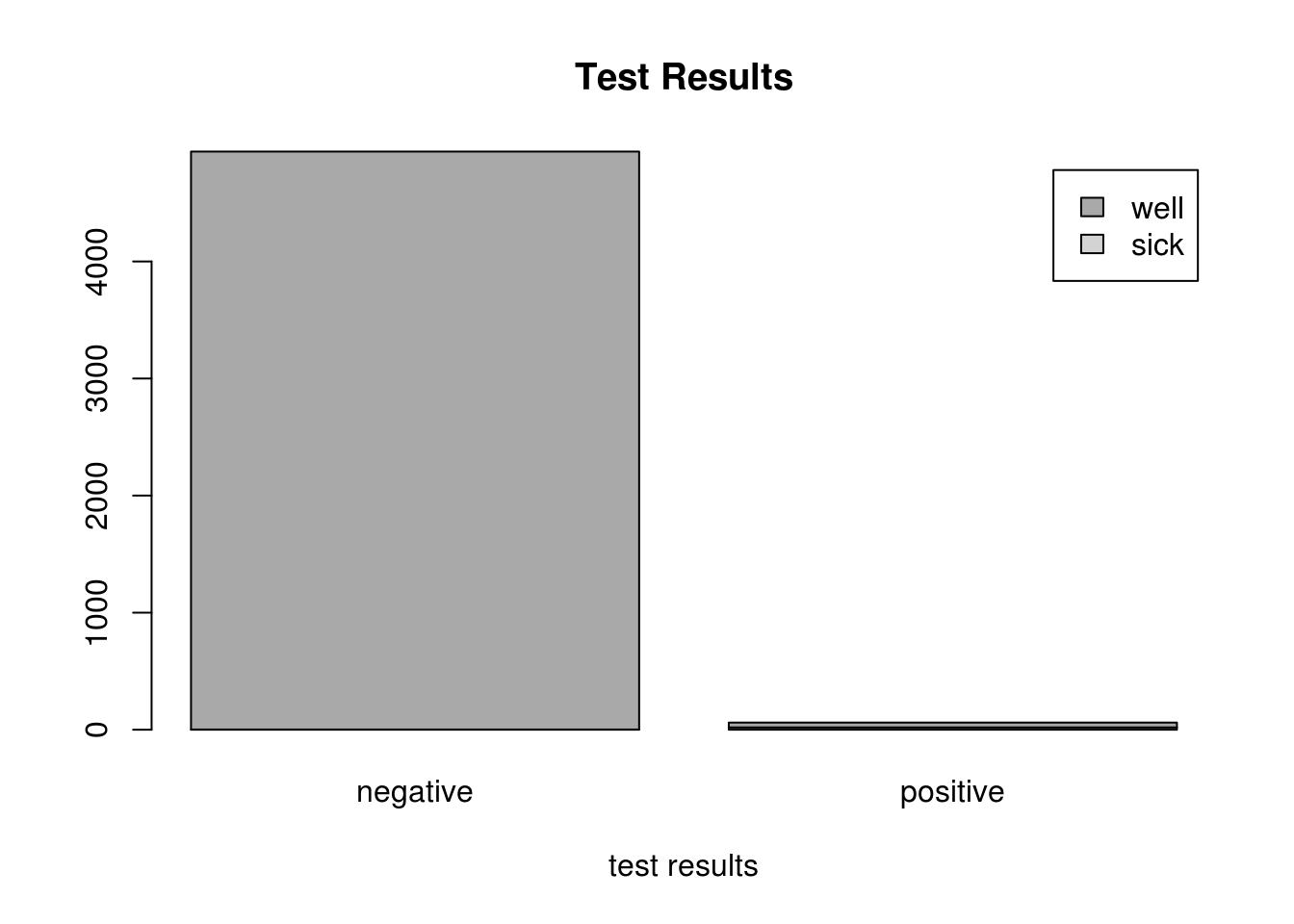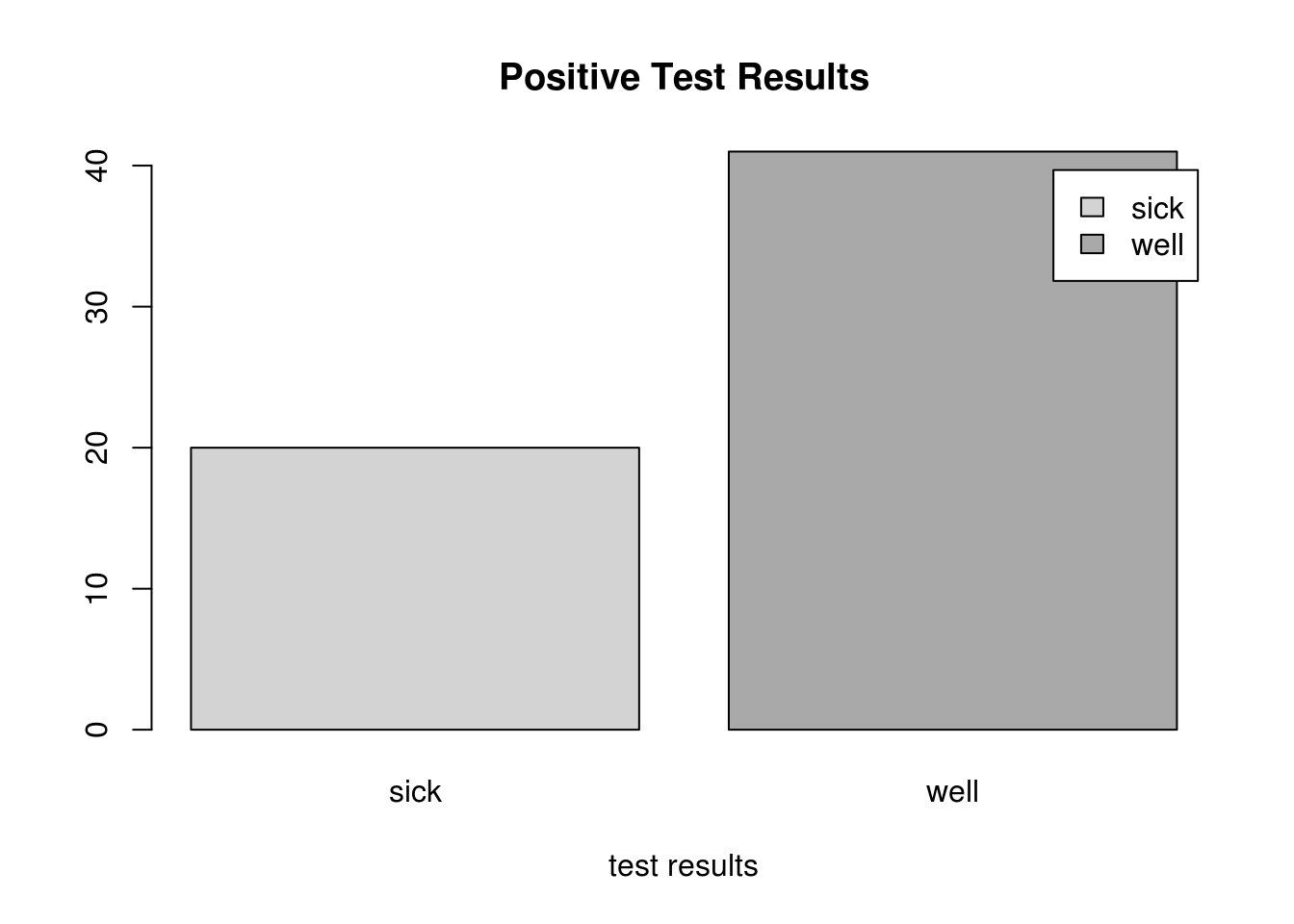## Intoduction

Problems in conditional probability often confound our common sense. Here’s an example concerning medical tests that can sometimes return ‘false positive’ results.

A laboratory blood test is 95 percent effective in detecting a certain disease when it is, in fact, present. However, the test also yields a “false positive” result for one percent of healthy persons. (That is, if a healthy person is tested, there is a 0.01 probability that they will test positive for the disease, even though they don’t really have it.) If 0.5 percent of the population actually has the disease, what is the probability that a person who has a positive test result actually has the disease?

Another way of asking the question: how worried should you be if your doctor phones and tells you the results are positive?

Before calculating the theoretical probabilities to answer the question, we can perform an experiment and actually see what happens. We don’t have access to real data that matches the problem situation, but we can simulate the data, randomly generating subjects that do or do not have the disease, and then randomly generating the results of their tests, based on the parameters given in the problem.

## Setting up the Simulation

First we decide how many trials to include in the simulation - let’s set up a MedicalTest dataframe with 5000 trials.

trial_limit <- 5000
trial <- 1:trial_limit
MedicalTest <- data.frame(trial)

Next, we make sure that our population has the disease in proportion to what was described in the problem.

MedicalTest$hasDisease <- (runif(trial_limit,0,1) <= 0.005) MedicalTest$health[MedicalTest$hasDisease] <- "sick" MedicalTest$health[!MedicalTest$hasDisease] <- "well" It is good to check and see if the randomizing that we did resulted in the expected proportions of subjects with and without the disease: t <- table(MedicalTest$hasDisease)
t <- round(prop.table(t) * 100, 2)
paste("disease free: ",t, "%", ", has disease: ",t, "%")
##  "disease free:  99.56 % , has disease:  0.44 %"

Next, we run the test: it will return positive results for 95% of those with the disease, and 1% of those without it.

for (i in trial) {
if (MedicalTest$hasDisease[i]) { MedicalTest$positive[i] <- (runif(1,0,1) <= 0.95)
} else {
MedicalTest$positive[i] <- (runif(1,0,1) <= 0.01) } } MedicalTest$results[MedicalTest$positive] <- "positive" MedicalTest$results[!MedicalTest$positive] <- "negative" ## Exploring the Simulation Looking at a bar plot of the responses, we can see that the positive and negative test results contain a mix of those subjects with and without the disease. Looks like a small number of well people received a positive test result, as expected. t <- table(MedicalTest$health, MedicalTest$results) barplot(t, main="Test Results", xlab="test results", col=c("light grey","dark grey"), legend = rownames(t))But we want to just restrict our attention to positive test results - a number that is dwarfed by the number of negative test results, since the disease is reasonably rare. We are looking at this from the perspective of someone who received a positive result: how worried should they be? Positives <- subset(MedicalTest, MedicalTest$positive)
t <- table(Positives$health) barplot(t, main="Positive Test Results", xlab="test results", col=c("light grey","dark grey"), legend = rownames(t))In our experimental simulated population, of the people that received a positive test result, the proportions of healthy and sick individuals are as follows. The percentage of people without the disease but yet received a postive test result may seem surprisingly high. t <- table(Positives$hasDisease)
t <- round(prop.table(t) * 100, 2)
paste("disease free: ",t, "%", ", has disease: ",t, "%")
##  "disease free:  67.21 % , has disease:  32.79 %"

## Direct Calculation

The problem can be expressed in terms of conditional probabilities. If we say that $$D$$ is the event that a person has the disease, and $$T$$ is the event that a person tests positive, then the event that the person has the disease, given that they have tested positive is $$D|T$$. For events $$A$$ and $$B$$, the formula for the probability of $$A|B$$ is:

$p(A|B) = \frac{p(A \cap B)}{p(B)}$

So we would like to find $$p(D|T)$$.

$p(D|T) = \frac{p(D \cap T)}{p(T)}$

First the probability of testing positive is the sum of the probability of testing positive with the disease.

$p(T)= p(T|D) \times p(D) + p(T|\neg D) \times p(\neg D)$

0.95*0.005 + 0.01*0.995
##  0.0147

$p(T)= 0.95 \times 0.005 + 0.01 \times 0.995 = 0.0147$

And the probability of testing positive and having the disease is

$p(D \cap T) = p(T \cap D) = p(T|D) \times p(D)$

0.95*0.005
##  0.00475

$p(D \cap T)= 0.95 \times 0.005 = 0.00475$

Finally

$p(D|T) = \frac{0.0475}{0.0147} = 0.323$

 0.00475/0.0147
##  0.3231293

So if you received a positive result there is only a 32.3% that you have the disease, and a 67.7% chance that you do not. This, hopefully, agrees reasonably well with what we found in our simulation. When thinking about these problems, we can be mislead by the very low probability of false positives. However, what we have to keep in mind is that (in this problem), most people do not have the disease: so the large number of healthy people, multiplied by the low probability of false positives, yields a significant portion of the overall positive results.